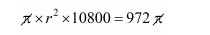# The diameter of a copper sphere is 18 cm.Question:

The diameter of a copper sphere is 18 cm. The sphere is melted and is drawn into a long wire of uniform circular cross-section. If the length of the wire is 108 m, find its diameter.

Solution:

The radius of copper sphere, $R=\frac{18}{2}=9 \mathrm{~cm}$

The volume of sphere $=\frac{4}{3} \pi \times(9)^{3}$

$=\frac{4}{3} \pi \times 729$

$=972 \pi \mathrm{cm}^{2}$

Since,

The sphere is melted and drawn into a long circular wire of length 108 m = 10800 cm

Let r be the radius of wire,

Clearly,

The volume of wire = volume of sphere$r^{2}=\frac{972}{10800}$

$=\frac{9}{100}$

$r=\frac{3}{10}$

$r=0.3 \mathrm{~cm}$

And, d = 2r = 0.6 cm

Hence, the diameter of the wire will be equals to $0.6 \mathrm{~cm}$